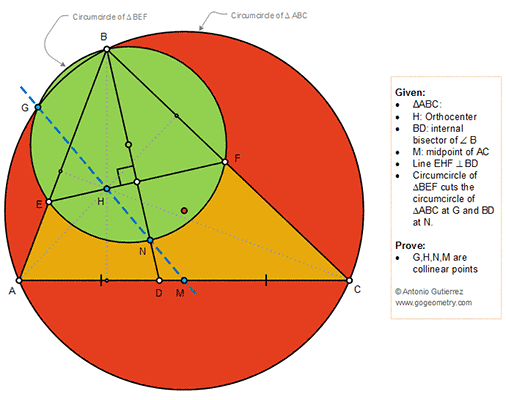## Wednesday, September 5, 2018

### Geometry Problem 1385: Triangle, Orthocenter, Circle, Circumcircle, Angle Bisector, Midpoint, Collinear Points

Geometry Problem. Post your solution in the comment box below.
Level: Mathematics Education, High School, Honors Geometry, College.

Details: Click on the figure below.#### 1 comment:

1.https://photos.app.goo.gl/Ki4AYSREXnaEaSAZ7

Let BD and BO extended meet circle O at P and Q
Let M is the midpoint of AC and NQ meet OP at M’
Let BM meet HO at R ( R is the centroid of triange ABC)
Note that HRO is the Euler line of triangle ABC
We have RO/RH= OM/BH= ½ (1) ( Property of Euler line)
Triangle BHQ simillar to OM’Q.. ( case AA)
So QO/QB= OM’/HB= ½ .. (2)
Compare (1) and (2) we have OM’=OM => M coincide to M’
So Q, H, N, M are collinear